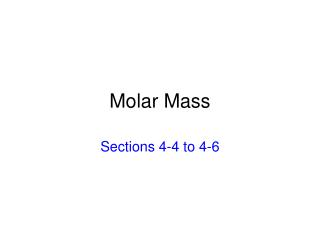DownloadDownload PresentationMolar Mass

# Molar Mass

Télécharger la présentation## Molar Mass

- - - - - - - - - - - - - - - - - - - - - - - - - - - E N D - - - - - - - - - - - - - - - - - - - - - - - - - - -
##### Presentation Transcript

1. Molar Mass Sections 4-4 to 4-6

2. Carbon • Atomic # is 6 • Atomic mass is 12.01 u • Molar mass (mass per mole) is 12.01 g/mole

3. Molar Mass • Molar mass for an element is found by taking the atomic mass for that element in the periodic table and changing the units to grams/mole. • Molar mass for a compound is found by adding the atomic masses (in grams) for ALL the elements in the compound.

4. H2O • Molar mass of hydrogen is 1.01g/mole • There are two atoms of hydrogen in water • Molar mass of oxygen is 16.0 g/mole 2(1.01 g/mole) H + 16.0 g/mole O = 18.02 g/mole H2O

5. NaCl • Molar mass of sodium is 22.99 g/mole • Molar mass of chlorine is 35.45 g/mole 22.99 g/mole Na + 35.45 g/mole Cl = 58.44 g/mole NaCl

6. What is the mass of 1.31 mol of silver nitrate? • Find the correct chemical formula for silver nitrate. silver = Ag+ nitrate = NO3- Silver nitrate is AgNO3

7. 2. Find the molar mass of AgNO3. molar mass Ag = 107.87 g/mole molar mass N = 14.01 g/mole molar mass O = 16.00 g/mole there are three atoms of O 107.87 g/mole Ag + 14.01 g/mole N + 3(16.00 g/mole O) = 169.88 g/mole AgNO3

8. Find the mass of 1.31 mole of AgNO3. X g AgNO3 169.88 g AgNO3 ______________ = ______________ 1.31 mole AgNO3 1 mole AgNO3 X = 222.54 g AgNO3

9. How many moles are in a 124 g sample of C8H18? • Find the molar mass of C8H18. molar mass C = 12.01 g/mole there are 8 atoms of C molar mass H = 1.01 g/mole there are 18 atoms of H 8(12.01 g/mole C) + 18(1.01 g/mole H) = 114.26 g/mole C8H18

10. 2. Find how many moles of C8H18 are in 124.0 grams. x moles C8H18 1 mole C8H18 _____________ = ____________ 124.0 g C8H18 114.26 g/mole C8H18 x = 1.09 moles C8H18

11. Copy the Molar Calculator from either the poster on the wall, or from page 108 in the text book. Do practice questions on page 109 # 4-8, 10-11, 13-16. These practice questions will be corrected in class on Thursday.